# Jensen formula

(diff) ← Older revision | Latest revision (diff) | Newer revision → (diff)

A relation connecting the values of a meromorphic function inside a disc with its boundary values on the circumference and with its zeros and poles. Letbe a meromorphic function in the disc; let,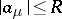, and,, be all the zeros and poles of, respectively, where each pole or zero is counted as many times as its order or multiplicity. If, then Jensen's formula holds:(1)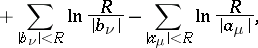in which the sums extend over all zeros and poles ofinside the disc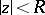; formula (1) was obtained by J.L. Jensen in . A small modification is necessary to adapt (1) to the case.

A more general formula holds, called by R. Nevanlinna the Poisson–Jensen formula, giving the values of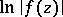at an arbitrary point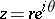other than a zero or a pole:(2)Formula (2) can be regarded as a generalization of the Poisson integral for a disc. Generalization of the Schwarz integral for the disc in exactly the same way gives the Schwarz–Jensen formula:(3)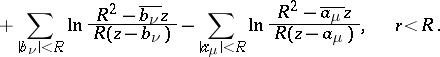Formulas of the type (1)–(3) can be constructed for half-planes and other domains. The formulas (1)–(3) play an important part in value-distribution theory.

A wide generalization of the formulas (1)–(3) has been obtained by M.M. Dzhrbashyan in his theory of classes of meromorphic functions (see ). He succeeded in obtaining a whole family of such formulas, depending on a certain continuous parameter,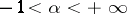, that is connected with an integro-differential operator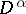; for example, formula (3) turns out to be the special case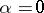.

Formulas (1) and (2) can be generalized for subharmonic functionsin a ball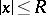in a Euclidean space,, as follows: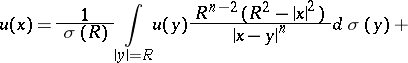(4)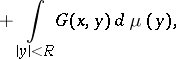where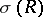is the area of the sphere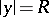in,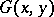is the Green function for the ball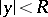with pole at, andis the positive measure associated with the subharmonic function. The first summand in (4) is the least harmonic majorant ofin the ball, expressed in the form of a Poisson integral over the boundary values; the second summand is a Green potential, which reduces in special cases to the logarithm of the modulus of the Blaschke product figuring in (2). Formula (2) is obtained from (4), taking into account that for a meromorphic function,is the difference of two subharmonic functions; formula (4) is applicable to functions of this type.

Now letbe a holomorphic function of several complex variables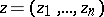,, in a closed polydisc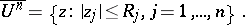Of great importance also is the Jensen inequality, which can be deduced easily from the properties of plurisubharmonic functions (cf. Plurisubharmonic function), and which forfollows immediately from formula (2):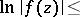(5)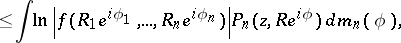where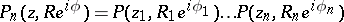is the Poisson kernel for, and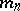is the normalized Haar measure on the distinguished boundary(see , ). Inequality (5), and certain higher-dimensional analogues of formula (2), find application in modern higher-dimensional value-distribution theory, see .

How to Cite This Entry:
Jensen formula. Encyclopedia of Mathematics. URL: http://encyclopediaofmath.org/index.php?title=Jensen_formula&oldid=17842
This article was adapted from an original article by E.D. Solomentsev (originator), which appeared in Encyclopedia of Mathematics - ISBN 1402006098. See original article